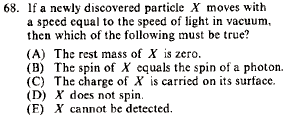GR 8677927796770177 | # Login | Register

GR8677 #68
Problem
 GREPhysics.NET Official Solution Alternate SolutionsSpecial Relativity}Relativistic Energy

The relativistic energy relation is,

As the speed of a particle , , since by the de Broglie relation, . Plug in the requirement into the energy relation to find that , as in choice (A).Alternate Solutions
 TimToolMan2018-04-12 16:27:04 We know that (using relativistic momentum and energy). Since we have which implies . And, from the relation , we can say that implies .Reply to this commentTimToolMan2018-04-04 16:21:50 Another way to think about it:\\\\r\\\\nTake if then and then which means cancelling like terms gives when Reply to this commentpranav2012-11-09 13:17:09 One more way of doing this is by recalling the following equation: The only way anyone (including Mr. Photon) can go at is by having a rest mass of zero. (For those who doubt this: The above equation can be re-written at v=c as: QED) Reply to this commentTimToolMan
2018-04-12 16:27:04
We know that (using relativistic momentum and energy). Since we have which implies . And, from the relation , we can say that implies .TimToolMan
2018-04-04 16:21:50
Another way to think about it:\\\\r\\\\nTake if then and then which means cancelling like terms gives when
 TimToolMan2018-04-12 16:28:04 Disregard this one, formatting mistake. See my other comment.Giubenez
2014-10-17 05:46:11
The easiest way is surely to compare it with the photon (that is known to have a rest mass = 0).
Since

and we know that photons have finite momentum the only possible explanation is that

().
Now our particle, having v =c must have m=0 to obtain a finite momentum..pranav
2012-11-09 13:17:09
One more way of doing this is by recalling the following equation:

The only way anyone (including Mr. Photon) can go at is by having a rest mass of zero.

(For those who doubt this:

The above equation can be re-written at v=c as:

QED)Astrophysicist
2011-10-02 11:34:45
One can also remember that photons(which travel at the speed of light) have rest mass = 0. Thus, this particle must have a rest mass = 0 as well.
 mpdude82012-04-19 15:15:23 You can't really say "particle X will automatically share the same characteristics of a photon" and deduce the answer from there, otherwise answer B also would be valid.
 deneb2018-10-10 04:47:41 The difference is that A MUST be true, so you have to pick A. Anything with v = c must be massless.shak
2010-07-31 21:20:43
Can we make a conclusion from this formula,
m=m(0)/(1-v^2/c^2)^0.5
then,
m(o)=m*(1-v^2/c^2)^0.5

when v approaches c, then m(0) goes to zero
Is this right?
thanks
 neon372010-11-03 11:55:06 umm....that looks correct to me. I cant think of an arguement to counter that (atleast yet).spacemanERAU
2009-10-20 08:37:41
Also, this problem is really simple if you remember that any particle that has speed of c MUST have rest mass of zero...no math involved2006-10-23 00:41:07
It should be
 spacemanERAU2009-10-18 18:35:28 agreed!The easiest way is surely to compare it with the photon (that is known to have a rest mass = 0). Since and we know that photons have finite momentum the only possible explanation is that (). Now our particle, having v =c must have m=0 to obtain a finite momentum..LaTeX syntax supported through dollar sign wrappers $, ex.,$\alpha^2_0$produces . type this... to get...$\int_0^\infty\partial\Rightarrow\ddot{x},\dot{x}\sqrt{z}\langle my \rangle\left( abacadabra \right)_{me}\vec{E}\frac{a}{b}\$